## Monday, April 8, 2013

### Using Color Calculators to Plot Patterns (TI-84+CSE, Casio Prizm)

We are in the age of color graphing calculators (and mobile apps). While the Casio Prizm and TI nSpire CX (with and without CAS) got the age started, it could be the next crop of color calculators, such as the TI-84+ Color Silver Edition that puts color into the mainstream.

These patterns are generated by using four colors and a function f(x,y) where

f(x,y) = remainder( int(Φ(x,y)) / 4)

with Φ(x,y) is a positive function. The value of f(x,y) determines on of four colors. I used the DRAWPATTERN (page 269 of the HP 39gii manual, 1st edition) as a template.

With the TI-84+ Color Silver Edition it takes about 40-45 minutes to complete. The real surprise (to me at least), was I did one with the Casio Prizm, which it took about six hours to complete; I was surprised there was any battery left. This is where the advantage of rechargeable battery of the TI-84+ CSE comes in. I am happy then to report that the battery of the TI-84+ CSE held up well.

Without any further ado, here are the graphics created! Nice way to spend a Sunday afternoon, I think.

With each picture I will list Φ(x,y). The window, except noted, is Xmin=-10, Xmax=10, Ymin=-10, Ymax=10.

Recall: f(x,y) = remainder( int(Φ(x,y)) / 4)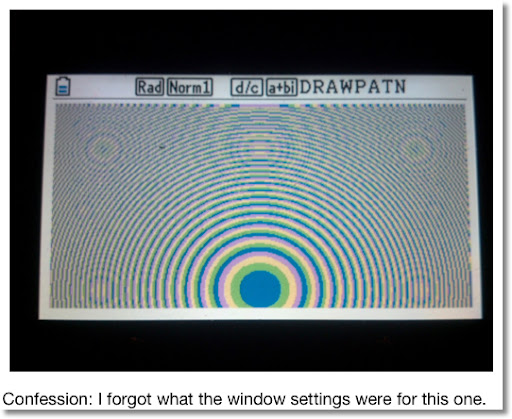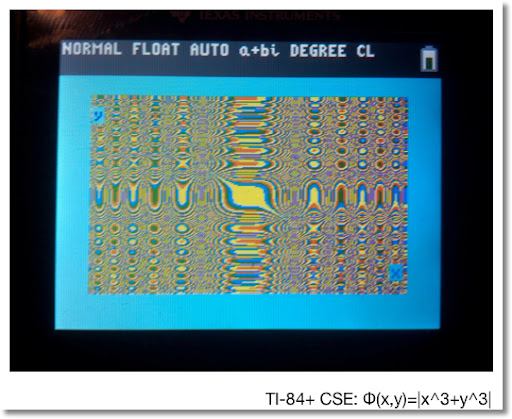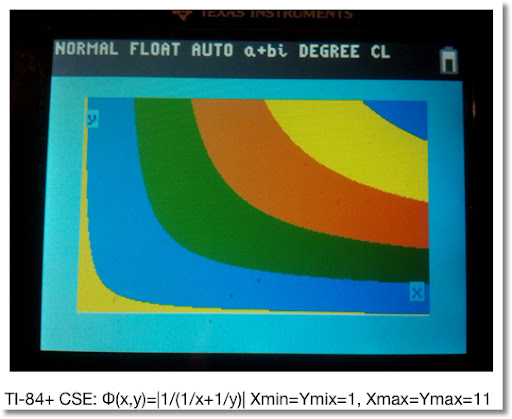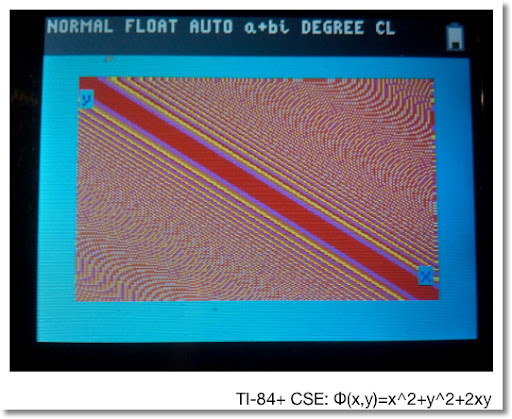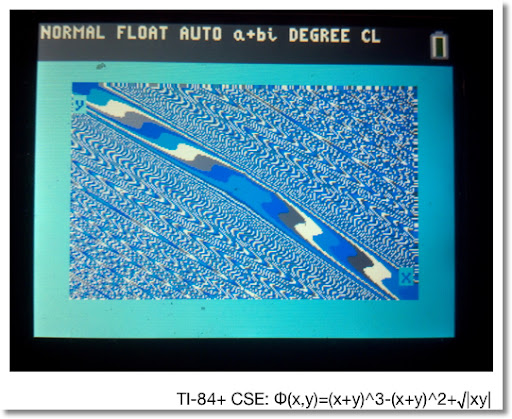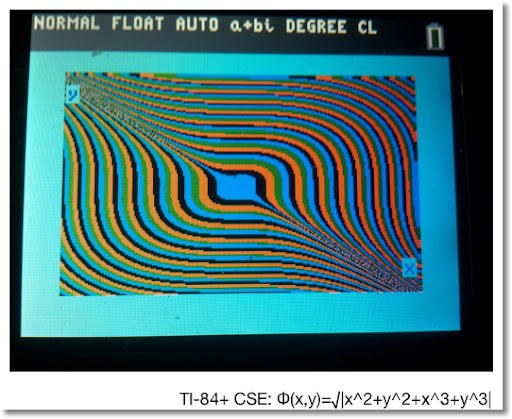Until next time,

Eddie

This blog is property of Edward Shore. 2013

### Casio fx-CG50: Sparse Matrix Builder

Casio fx-CG50: Sparse Matrix Builder Introduction The programs can create a sparse matrix, a matrix where most of the entries have zero valu...